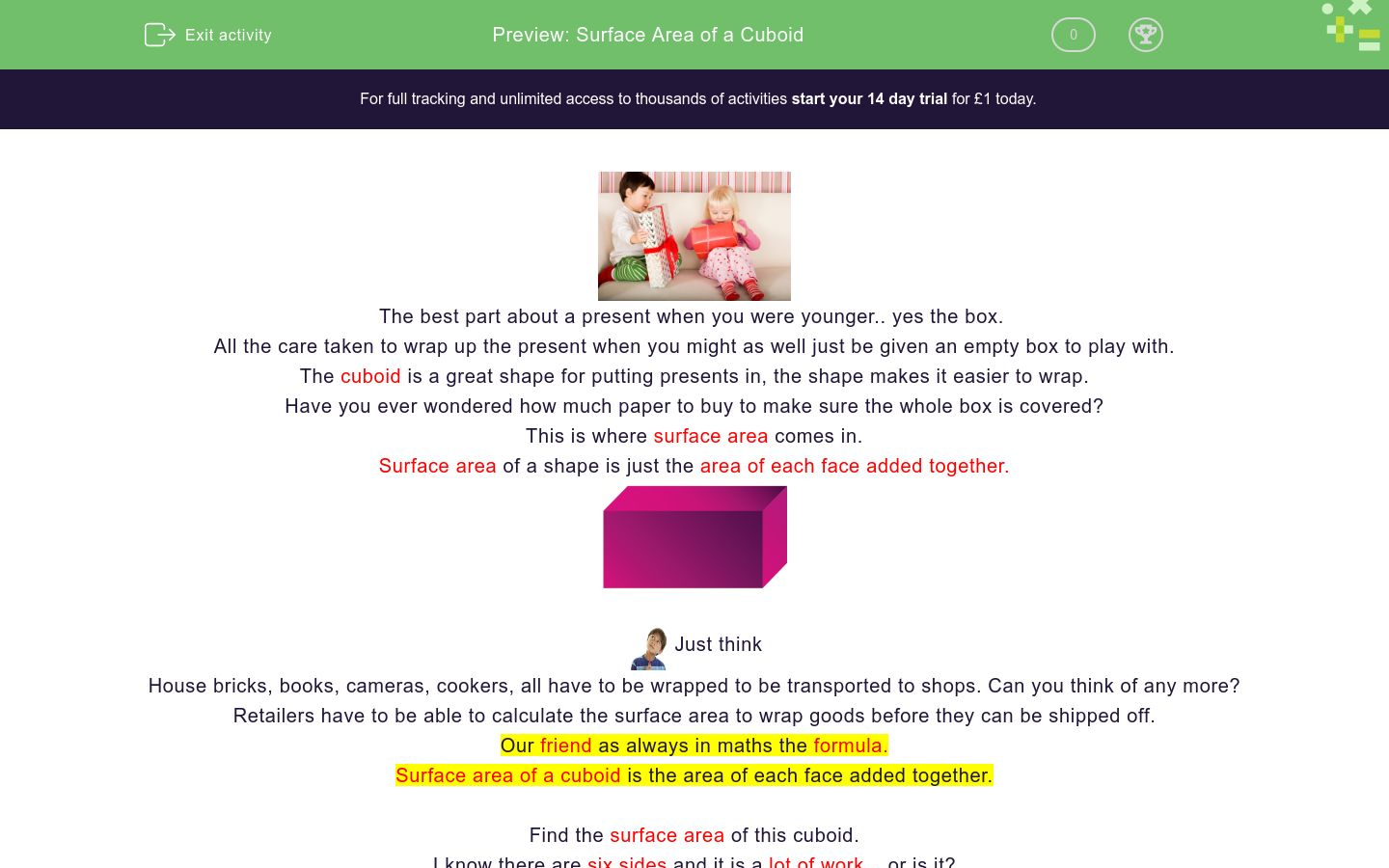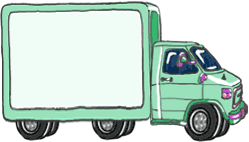# Surface Area of a Cuboid

In this worksheet students will learn the formula for finding the surface area of a cuboid. They will be able to find the surface area of cuboids and solve simple problems.Key stage:  KS 4

GCSE Subjects:   Maths

GCSE Boards:   AQA, Eduqas, Pearson Edexcel, OCR

Curriculum topic:   Geometry and Measures, Mensuration

Curriculum subtopic:   Mensuration and Calculation, Volume and Surface Area Calculations

Difficulty level:### QUESTION 1 of 10The best part about a present when you were younger.. yes the box.

All the care taken to wrap up the present when you might as well just be given an empty box to play with.

The cuboid is a great shape for putting presents in, the shape makes it easier to wrap.

Have you ever wondered how much paper to buy to make sure the whole box is covered?

This is where surface area comes in.

Surface area of a shape is just the area of each face added together.Just think

House bricks, books, cameras, cookers, all have to be wrapped to be transported to shops. Can you think of any more?

Retailers have to be able to calculate the surface area to wrap goods before they can be shipped off.

Our friend as always in maths the formula.

Surface area of a cuboid is the area of each face added together.

Find the surface area of this cuboid.

I know there are six sides and it is a lot of work... or is it?

Us mathematicians are always looking for a short cut if possible.

Look at the break down below.Surface Area

Find the area of the face we are looking directly at.   5 x 4 = 20 cm²

There is another face exactly the same on the opposite side of the cuboid.  No working out necessary we have already done it.

We now have Face 1 = 20 cm²

Face 2 = 20 cm²

Now let us look at the face on the right hand side and work out the area.  3 x 5 = 15 cm²

The opposite face is also the same.

We now have Face 1 = 20 cm²

Face 2 = 20 cm²

Face 3 = 15 cm²

Face 4 = 15 cm²

Now find the remaining two faces in the same way.  4 x 3 = 12 cm²

We now have Face 1 = 20 cm²

Face 2 = 20 cm²

Face 3 = 15 cm²

Face 4 = 15 cm²

Face 5 = 12 cm²

Face 6 = 12 cm²

Total surface area   = 94 cm²

And remember measurement to do with area is measured in units squared.

Happy wrapping.What is the surface area of this of this cuboid?

280 cm²

156 cm²

320 cm²

180 cm²

Match up

## Column B

Surface Area of cuboid W = 4 cm , L = 5 cm, H = 2 ...
104 cm²
Surface Area cuboid W = 4 cm , L = 5 cm, H = 3 cm
94 cm²
Surface Area of cuboid W = 6 cm , L = 5 cm, H = 2 ...
76 cm²This tool box has dimensions W 30 cm, L 10 cm, H  20 cm

## Column B

Surface Area of cuboid W = 4 cm , L = 5 cm, H = 2 ...
104 cm²
Surface Area cuboid W = 4 cm , L = 5 cm, H = 3 cm
94 cm²
Surface Area of cuboid W = 6 cm , L = 5 cm, H = 2 ...
76 cm²The dimensions of this cuboid are W = 5 cm, L = 8 cm, H = 2 cm.

Calculate the surface area.What is the surface area of this cube with side 4 cm?Calculate the surface area of this cuboid.

184 cm²

136 cm²

163 cm²

148 cm²What is the difference between the surface area of these cubes?

One has side 5 cm and the other 3 cm?

184 cm²

136 cm²

163 cm²

148 cm²Calculate the surface area of this juice carton.

Dimensions are L = 4 cm, W = 2 cm and H = 5 cm

68 cm²

82 cm²

58 cm²

76 cm²

What do you have to do, in order to find the surface area of a cuboid?

Surface area is used a great deal in the packaging industry. To calculate the surface area you have to find the area of all the faces of a cuboid and then add the answers together. It is best to write things down methodically as you go along.What is the surface area of the wagon of this lorry?

L = 5.5 m, W = 1.5 m, H = 2.5 m

• Question 1What is the surface area of this of this cuboid?

320 cm²
EDDIE SAYS
The only way to get to grips with these questions is to do them methodically. Find the area of one face 12 x 4 = 48 12 x 4 = 48 Then find the next two 4 x 7 = 28 4 x 7 = 28 And finally the remaining two 12 x 7 = 84 12 x 7 = 84 The total them all up 320 cm² Look out for a top tip in the next activity.
• Question 2

Match up

## Column B

Surface Area of cuboid W = 4 cm ,...
76 cm²
Surface Area cuboid W = 4 cm , L ...
94 cm²
Surface Area of cuboid W = 6 cm ,...
104 cm²
EDDIE SAYS
Are you able to use one of the shorter ways to calculate these. It is just a matter of practice. 4 x 5 = 20 x 2 = 40 4 x 2 = 8 x 2 = 16 5 x 2 = 10 x 2 = 20 total surface area = 76 cm² 4 x 5 = 20 x 2 = 40 4 x 3 = 12 x 2 = 24 3 x 5 = 15 = 30 total surface area = 94cm² 6 x 5 = 30 x 2 = 60 6 x 2 = 12 x 2 = 24 5 x 2 = 10 x 2 = 20 Total surface area = 104 cm²
• Question 3This tool box has dimensions W 30 cm, L 10 cm, H  20 cm

EDDIE SAYS
If in doubt sketch it out I always say. If you can't visualise this without the numbers on. Sketch out a cuboid, add the numbers and work out the area of the faces from there. Or use the top tip from the previous question. 20 x 10 = 200 + 200 (for two faces) 30 x 20 = 600 + 600 (for two faces) 30 x 10 = 300 + 300( for two faces) Total surface area = 400 + 1200 + 600 = 220 cm² This is the beauty of maths. You can set things out in various ways.
• Question 4The dimensions of this cuboid are W = 5 cm, L = 8 cm, H = 2 cm.

Calculate the surface area.

132
EDDIE SAYS
This is a little tricky because the numbers are not on the diagram. Top tip - make sure that each number is multiplied by a different number twice. 5 x 8 = 40 5 x 8 = 40 (two opposite faces) 5 x 2 = 10 5 x 2 = 10 (two opposite faces) 2 x 8 = 16 2 x 8 = 16 (two opposite faces) 40 + 40 + 10 + 10 + 16 + 16 = 132 cm² In someways it is easier that working out what to multiply from looking at a diagram.
• Question 5What is the surface area of this cube with side 4 cm?

EDDIE SAYS
This is such a gift on an exam paper. Why I hear you ask. Not much work. We know a square has sides all the same. The area of the faces are all the same too. so 4 x 4 = 16 cm² There are 6 sides to a cube 16 x 6 = 96 cm² Magic.
• Question 6Calculate the surface area of this cuboid.

184 cm²
EDDIE SAYS
Hopefully you are getting the hang of this now. 5 x 4 = 20 x 2 = 40 5 x 8 = 40 x 2 = 80 4 x 8 = 32 x 2 = 64 Total surface area = 136 cm² Unfortunately there is quite a bit of work to these so hang on in there.
• Question 7What is the difference between the surface area of these cubes?

One has side 5 cm and the other 3 cm?

EDDIE SAYS
Thank goodness we are working from a cube otherwise this would take forever. We know a square has sides all the same. The area of the faces are all the same too. so 5 x 5 = 25 cm² There are 6 sides to a cube 25 x 6 = 150 cm² 3 x 3 = 9 cm² 9 x 6 = 54 cm² 150 - 54 = 96 cm²
• Question 8Calculate the surface area of this juice carton.

Dimensions are L = 4 cm, W = 2 cm and H = 5 cm

76 cm²
EDDIE SAYS
Thirsty work isn't it? Again it is just working it out in the method that suits you. Mine preferred method is this way. 4 x 2 = 8 x 2 = 16 4 x 5 = 20 x 2 = 40 2 x 5 = 10 x 2 = 20 Add them all up and hey presto 76 cm²
• Question 9

What do you have to do, in order to find the surface area of a cuboid?

Surface area is used a great deal in the packaging industry. To calculate the surface area you have to find the area of all the faces of a cuboid and then add the answers together. It is best to write things down methodically as you go along.
EDDIE SAYS
It really is all you have to do. It can sometimes be a lot of work. Look out in the explanations that follow for ways to make things a little quicker.
• Question 10What is the surface area of the wagon of this lorry?

L = 5.5 m, W = 1.5 m, H = 2.5 m

51.5
EDDIE SAYS
Decimals. Don't you just love them? Treat them in the same way. 5.5 x 1.5 = 8.25 x 2 = 16.5 5.5 x 2.5 = 13.25 x 2 =27.5 1.5 x 2.5 = 3.75 x 2 = 7.5 And now just add them up.
---- OR ----

Sign up for a £1 trial so you can track and measure your child's progress on this activity.

### What is EdPlace?

We're your National Curriculum aligned online education content provider helping each child succeed in English, maths and science from year 1 to GCSE. With an EdPlace account you’ll be able to track and measure progress, helping each child achieve their best. We build confidence and attainment by personalising each child’s learning at a level that suits them.

Get started